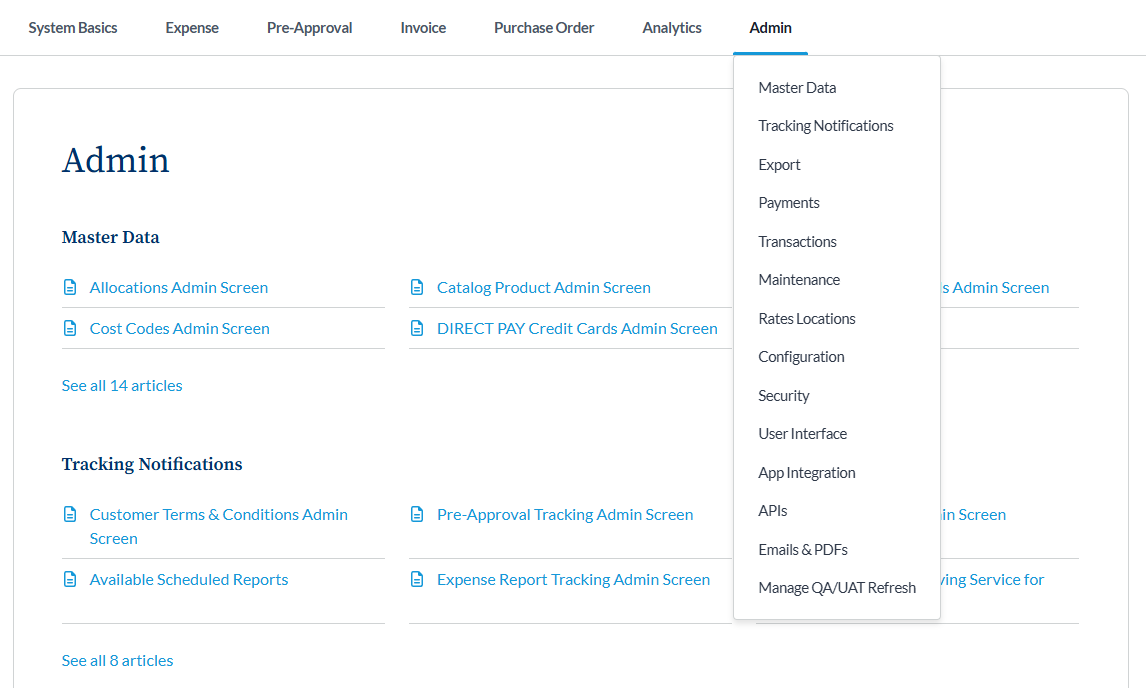The following resources will help new admins quickly get up to speed on Emburse Chrome River.

## Environments

Understanding Instances and Environments

Get Started in the QA/UAT Environment

## System Status

Help Desk System Status Dashboard

## Support

How to Create a Help Desk Case

Help Desk Approved Contacts List

How to Subscribe to Release Notes

## Help Center

Help Center articles for admins are organized in the same order that each screen appears inside Chrome River.<% if (allItems.length > 1) { %>

<% } %>
<% var getColumnClasses = function(numberColumns) { var classNames = 'col-12'; if (numberColumns >= 2) classNames += ' md:col-6'; if (numberColumns >= 3) classNames += ' lg:col-4'; if (numberColumns >= 4) classNames += ' xl:col-3'; return classNames; } %>

##<%= heading %>

<% } %>
<% var getColumnClasses = function(numberColumns) { var classNames = 'col-12'; if (numberColumns >= 2) classNames += ' md:col-6'; if (numberColumns >= 3) classNames += ' lg:col-4'; if (numberColumns >= 4) classNames += ' xl:col-3'; return classNames; } %>

##<%= heading %>

<% } %>
<% var maxSections = 5 %>
<% if (sections.length) { %>
<% sections.forEach(function(section) { %>
• <%= partial('partial-article-list-sections', { id: 'section-' + section.id, parentId: '#' + id, sections: section.sections, activeCategoryId: activeCategoryId, activeSectionId: activeSectionId, activeArticleId: activeArticleId, partial: partial }) %> <% if (section.articles.length) { %> <% } %>
• <% }); %>
<% } %>
<% if (sections.length) { %>
<% sections.slice(0, maxSections).forEach(function(section) { %>
• <%= section.name %> <%= partial('partial-section-list-sections', { parent: section, sections: section.sections, maxSections: maxSections, partial: partial }) %>
• <% }); %> <% if (sections.length > maxSections) { %>
• See more
• <% } %>
<% } %>
<% var getColumnClasses = function(numberColumns) { var classNames = numberColumns === 'auto' ? 'col-auto' : 'col-12'; if (numberColumns >= 2) classNames += ' md:col-6'; if (numberColumns >= 3) classNames += ' lg:col-4'; if (numberColumns >= 4) classNames += ' xl:col-3'; return classNames; } %>
<% blocks.forEach(function(block, index) { %>
• <% if (imageHeight) { %><% } %>
<% if (block.name) { %>

<% if (block.html_url) { %> <%= block.name %> <% } else { %> <%= block.name %> <% } %>

<% } %> <% if (block.description) { %>

<%= block.description %>

<% } %>
• <% }) %>